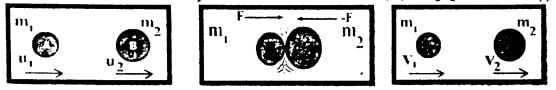What is the law of conservation of momentum?

OR

State and explain the law of conservation of momentum?

Difficulty: Hard

Law of conservation of momentum:

The momentum of an isolated system of two or, more two interacting bodies remains constant.

Example:

Consider the example of an air-filled balloon as described under the third law of motion. In this case, the balloon and the air inside it form a system. Before releasing the balloon, the system was at rest and hence the initial momentum of the system was zero. As soon as the balloon is set free, air escapes out of it with some velocity. The air coming out of it possesses momentum. To conserve momentum, the balloon moves in a direction opposite to that of air rushing out.

Explanation:

Law of conservation of momentum applies to all objects in the universe. A rocket and jet engine are taking off. The recoil of a gun is an example that demonstrates the importance of the law of conservation of momentum.

Case I:

Consider an isolated system of two spheres of masses m1 and m2. They are moving in a straight line with initial velocities u1 and u2 respectively such that u1 is greater than u2. The sphere of mass m1 approaches the sphere of mass m2 as they mThe initial

The initial momentum of mass m1 =m1u1

The initial momentum of mass m2 =m2u2

The total initial momentum of the system before collision = m1u1+m2u2 ………(i)Case II:

After sometimes mass m1 hit m2 with some force. According to newton's third law of motion, m2 exerts an equal and opposite reaction force on m1. Let their velocities become v1 and v2 respectively after the collision. Then

Final momentum of mass m1 = m1u1

Final momentum of mass m2 = m2u2

Total final momentum of the system before collision = m1u1+m2u2 ………(ii)

According to the Law of conservation of momentum:

[ total initial momentum of the system before collison] = [ total initial momentum of the system before system]

m1u1 + m2u2 = m1v1 + m2v2......... (iii)

Equation (iii) shows that the momentum of an isolated system before and after collisions remains the same which is the law of conservation of momentum. The Law of conservation of momentum is important and has vast applications.